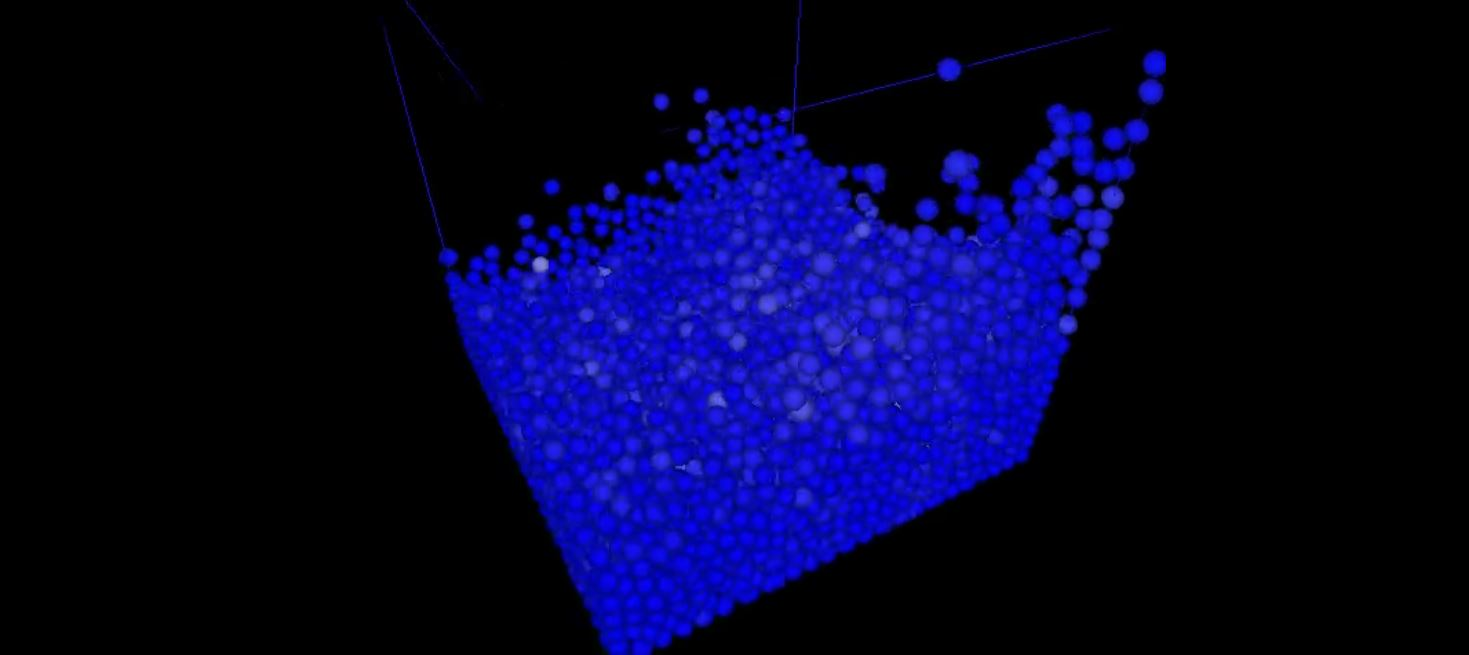# Fluid Simulation in Computer GraphicsSmoothed particle hydrodynamics, SPH, is a method that was first implemented in 1977 for astrophysical simulations by Gingold et al. Since then, SPH has become a popular method for complex fluid simulations. SPH is a mesh-free Lagrangian method where the fluid is split into discrete sets defined as particles, which move in space and change physical properties as time progresses. In the video below you can follow our implementation of a Divergence-Free SPH fluid simulation in computer graphics, based on the publication from Jan Bender et al.

``````function performFluidSimulation {
for all particles i do // Init
find neighbourhoods Ni(0)
for all particles i do //Init pi and ai
compute densities ρi(0)
compute factors αi(0)
while (t < tmax) do // Start simulation
for all particles i do //Predict velocities vi∗
vi = vi + ∆tFg=mi
correctDensityError(a, vi) // Fulfill ρ∗ − ρ0 = 0
for all particles i do // Update positions
xi(t + ∆t) = xi(t) + ∆tvi∗
for all particles i do // Update neighbourhoods
find neighbourhoods Ni(t + ∆t)
for all particles i do // Update ρi and αi
compute densities ρi(0)
compute factors αi(0)
correctDivergenceError(α, v*) // Fulfill Dρ / Dt = 0
}
``````

One of the major challenges was to get our pressure solver to work correctly and efficiently. Since the pressure force is dependant on a particle’s neighbours velocities and densities, a method to efficiently sorting through particles is required. The difference with the method from Jan Bender et al. compared to other SPH fluid simulation methods is that two distinct solvers are to minimise the divergence and density error whereas most other methods uses only one. This allows for fast convergence which leads to fewer iterations.

A report discussing the method in depth can be found here and the code is available on github.